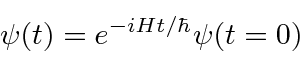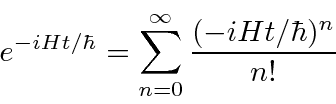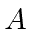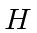## More Fun with Operators

We find the time development operator by solving the equation.This implies thatis the time development operator. In some cases we can calculate the actual operator from the power series for the exponential.We have been working in what is called the Schrödinger picture in which the wavefunctions (or states) develop with time. There is the alternate Heisenberg picture in which the operators develop with time while the states do not change. For example, if we wish to compute the expectation value of the operatoras a function of time in the usual Schrödinger picture, we getIn the Heisenberg picture the operator.

We use operator methods to compute the uncertainty relationship between non-commuting variableswhich gives the result we deduced from wave packets forand.

Again we use operator methods to calculate the time derivative of an expectation value.(Most operators we use don't have explicit time dependence so the second term is usually zero.) This again shows the importance of the Hamiltonian operator for time development. We can use this to show that in Quantum mechanics the expectation values forandbehave as we would expect from Newtonian mechanics (Ehrenfest Theorem).Any operatorthat commutes with the Hamiltonian has a time independent expectation value. The energy eigenfunctions can also be (simultaneous) eigenfunctions of the commuting operator. It is usually a symmetry of thethat leads to a commuting operator and hence an additional constant of the motion.

Jim Branson 2013-04-22